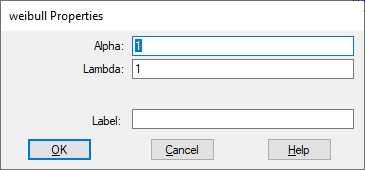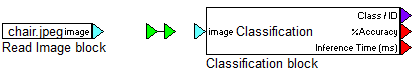## polyRoots

Block Category: Matrix Operation

Input: Coefficients vector starting with the coefficient of the highest order term at the top.

Outputs: Two vectors containing the real and corresponding imaginary parts of the roots.

Description: The polyRoots block finds the roots of polynomials with real coefficients.

#### Example

The following diagram finds the real and imaginary roots for the polynomial: